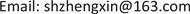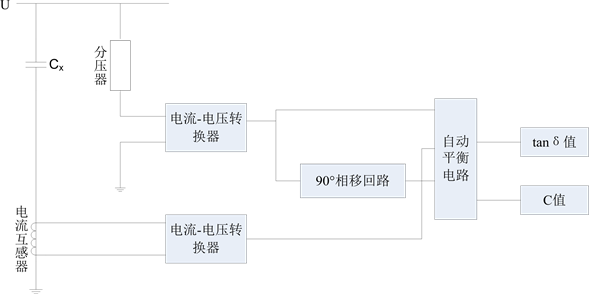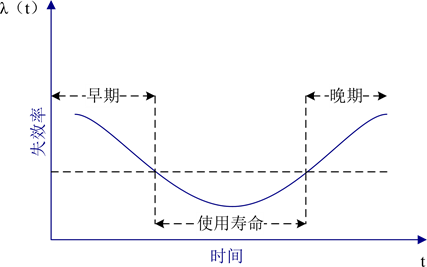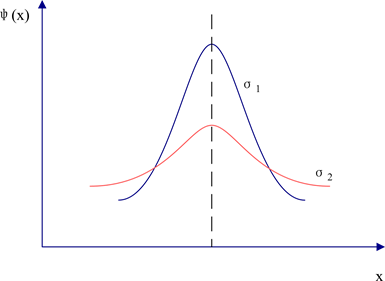﻿ 基于电子式互感器在线监测数据的绝缘诊断技术研究 Research on the Insulation Diagnosis Technology Based on the On-Line Monitoring Data of Electronic Transformers

Smart Grid
Vol.07 No.06(2017), Article ID:23187,7 pages
10.12677/SG.2017.76056

Research on the Insulation Diagnosis Technology Based on the On-Line Monitoring Data of Electronic Transformers

Xin Zheng, Jun Li, Ming Lei, Lieqi Yan

State Grid Hubei Electric Power Company Measuring Center, Wuhan HubeiReceived: Dec. 5th, 2017; accepted: Dec. 19th, 2017; published: Dec. 27th, 2017ABSTRACT

At present, the electronic transformer has been used in large scale because of its excellent performance. State Grid Corp will strive to solve the problem of reliability and accuracy of digital measurement in the “13th Five-Year” period. On-line monitoring system of the digital measurement primary equipment is a kind of methods measuring the insulation condition of equipment. And the deployment of the on-line monitoring system was lack of statistical judgment for the data collection before, so that the real-time situation of a device cannot be mastered by monitoring data. In this paper, we will focus on a kind of insulation diagnosis technology based on on-line monitoring data, and use the principle of statistical analysis to determine the insulation and life prediction of the equipment.

Keywords:Electronic Instrument Transformer, On-Line Monitoring, Dielectric Loss, Expert Analysis System, Efficiency of Loss1. 引言

2. 电子式互感器在线监测现状

3. 绝缘类监测对象

3.1. 介质损耗的表示方法

$\text{tan}\delta =\frac{p}{Q}$ (1)

3.2. 介质损耗的监测原理Figure 1. Schematic diagram of tanδ and C of dielectric dissipation

3.3. 系统测量精度的相关问题

3.3.1. 系统谐波

3.3.2. 门电路的延时

3.3.3. 过零点的漂移

4. 绝缘诊断技术

4.1. 绝缘诊断技术概述

4.2. 现场测试数据

4.3. 建立数学模型

$F\left(t\right)=P\left(T\le t\right)$ (2)

$\stackrel{¯}{t}=\frac{1}{n}\underset{i=1}{\overset{n}{\sum }}{t}_{i}$ (3)

$\lambda \left(t\right)=\underset{{}_{\Delta }t\to 0}{\mathrm{lim}}\frac{1}{{}_{\Delta }t}\cdot P,\text{\hspace{0.17em}}\text{\hspace{0.17em}}在\left(t,t+{}_{\Delta }t\right)内失效$ (4)

$\frac{{}_{\Delta }n}{N-n\left(t\right)}=\frac{在时间\left(t,t+{}_{\Delta }t\right)内失效的产品数}{在时刻t仍正常工作的产品数}$ (5)Figure 2. Schematic diagram of product failure rate

4.4. 概率统计分析

$\stackrel{¯}{\xi }=\frac{1}{n}\underset{i=1}{\overset{n}{\sum }}{X}_{i}$ (6)

$\phi \left(x\right)=\frac{1}{\sqrt{2\text{π}}\sigma }{\text{e}}^{-\frac{{\left(x-a\right)}^{2}}{2\sigma }}$ (7)

4.5. 实际案例

${P}_{r}={C}_{n}^{r}×{P}^{r}×{\left(1-P\right)}^{n-r}$ (8)

${P}_{2}={C}_{10}^{2}×{0.08}^{2}×{\left(1-0.08\right)}^{10-2}=0.147$ (9)

${P}_{3}={C}_{10}^{3}×{0.08}^{3}×{\left(1-0.08\right)}^{10-3}=0.035$ (10)Figure 3. Schematic diagram of σ

5. 总结

Research on the Insulation Diagnosis Technology Based on the On-Line Monitoring Data of Electronic Transformers[J]. 智能电网, 2017, 07(06): 507-513. http://dx.doi.org/10.12677/SG.2017.76056

1. 1. 陈应林, 黄德祥. 数字式电能计量系统及检定装置设计[J]. 电力自动化设备, 2009, 29(4): 114-117.

2. 2. Basak, A. (1999) Condition Monitoring of Power Transformers. Engineering Science & Education Journal, 8, 41-46. https://doi.org/10.1049/esej:19990107

3. 3. 佟继春. 用于变压器油中多种溶解气体在线监测的智能化传感器研究[D]: [硕士学位论文]. 重庆: 重庆大学, 2004.

4. 4. 白维, 曾成碧, 王涛. 高压电气设备绝缘在线监测的研究[J]. 中国测试技术, 2006, 32(1): 288-288.

5. 5. 陈季丹. 电介质物理学[M]. 北京: 机械工业出版社, 1982.

6. 6. 李国庆, 庄重, 王振浩. 电容型电气设备介质损耗角的在线监测[J]. 电网技术, 2007, 31(7): 55-58+68.

7. 7. 董垠峰. 零点漂移的原理及处理方式[J]. 盐业与化工, 2015, 44(8): 41-44.

8. 8. 严璋, 黄鞠铭. 绝缘诊断技术的新动向——第三届中日绝缘诊断技术讨论会侧记[J]. 高电压技术, 1995(1): 90-92.

9. 9. 汤胜道, 汪凤泉. 失效率随时间而变的n中取k表决系统可靠性分析[J]. 系统工程学报, 2005 20(5): 555-558.

10. 10. 米特洛波尔斯基. 正态分布[M]. 北京: 科学出版社, 1959.